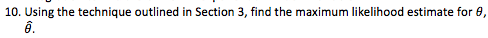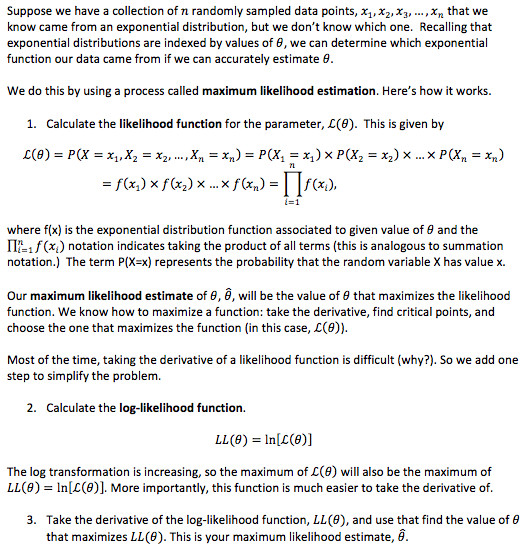# 10. Using the technique outlined in Section 3, find the maximum likelihood estimate for 0, Suppose we have a collection of n randomly sampled data points, x, x,X3,...,Xn that weknow came from an exponential distribution, but we don't know which one. Recalling thatexponential distributions are indexed by values of , we can determine which exponentialfunction our data came from if we can accurately estimate 0We do this by using a process called maximum likelihood estimation. Here's how it works.1. Calculate the likelihood function for the parameter, L(0). This is given byf(x) x f(x) x... x f(x) =f(x,),=1where f(x) is the exponential distribution function associated to given value of e and theIP xnotation indicates taking the product of all terms (this is analogous to summationnotation.) The term P(X-x) represents the probability that the random variable X has value x.Our maximum likelihood estimate of 8,, will be the value of 0 that maximizes the likelihoodfunction. We know how to maximize a function: take the derivative, find critical points, andchoose the one that maximizes the function (in this case, L(e)).Most of the time, taking the derivative of a likelihood function is difficult (why?). So we add onestep to simplify the problem.2. Calculate the log-likelihood functionLL(e) In[(e)]The log transformation is increasing, so the maximum of L(e) will also be the maximum ofLL(0) In[(0)]. More importantly, this function is much easier to take the derivative of.3. Take the derivative of the log-likelihood function, LL (0), and use that find the value of ethat maximizes LL(0). This is your maximum likelihood estimate, ê

Question
3 views

The first picture is the actual question i have to answer using section 3 which is shown in the second photo. Clean legible handwriting would be very much appreciated as i have already recieved some answers i can not read or understandhelp_outlineImage Transcriptionclose10. Using the technique outlined in Section 3, find the maximum likelihood estimate for 0, fullscreenhelp_outlineImage TranscriptioncloseSuppose we have a collection of n randomly sampled data points, x, x,X3,...,Xn that we know came from an exponential distribution, but we don't know which one. Recalling that exponential distributions are indexed by values of , we can determine which exponential function our data came from if we can accurately estimate 0 We do this by using a process called maximum likelihood estimation. Here's how it works. 1. Calculate the likelihood function for the parameter, L(0). This is given by f(x) x f(x) x... x f(x) =f(x,), =1 where f(x) is the exponential distribution function associated to given value of e and the IP xnotation indicates taking the product of all terms (this is analogous to summation notation.) The term P(X-x) represents the probability that the random variable X has value x. Our maximum likelihood estimate of 8,, will be the value of 0 that maximizes the likelihood function. We know how to maximize a function: take the derivative, find critical points, and choose the one that maximizes the function (in this case, L(e)). Most of the time, taking the derivative of a likelihood function is difficult (why?). So we add one step to simplify the problem. 2. Calculate the log-likelihood function LL(e) In[(e)] The log transformation is increasing, so the maximum of L(e) will also be the maximum of LL(0) In[(0)]. More importantly, this function is much easier to take the derivative of. 3. Take the derivative of the log-likelihood function, LL (0), and use that find the value of e that maximizes LL(0). This is your maximum likelihood estimate, ê fullscreen
check_circle

Step 1

Introduction:

The exponentially distributed random variable X has parameter θ.

The probability density function (pdf) of X is:

f (x) = θ eθx; x > 0; θ > 0.

A random sample of siz...

### Want to see the full answer?

See Solution

#### Want to see this answer and more?

Solutions are written by subject experts who are available 24/7. Questions are typically answered within 1 hour.*

See Solution
*Response times may vary by subject and question.
Tagged in

### Statistics